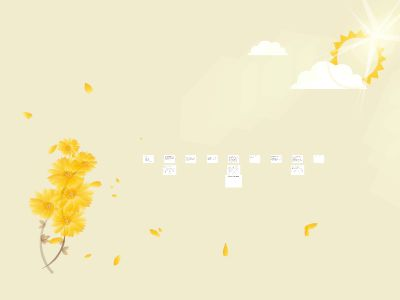Publications: 131 | Followers: 0

## Design of a 2 band antenna - arrlwcf.org

Publish on Category: Birds 0

Design of a 2 band antenna
It’s well known how to design an antenna to work on 2 bands:Insert traps resonant at the higher frequency so that the inner part is a half wavelength at the higher frequencyBut the “standard” means that on the higher frequency only part of the antenna is used.We also know how to make a short (less than ½ wavelength) antenna work – put in loading coils to bring the antenna to resonanceImplication is that if we had an antenna that was too long (!) we can bring it to resonance with loading capacitors
Why not combine the two ideas to make the loading work both ways? But how?Answer is parallel L-C trap with a resonant frequency somewhere between the two bands we’d like the antenna to work on.L-C trap reactance is inductive below its resonant frequency and capacitive above the resonant frequency.Need a procedure to calculate the L-C values to make everything come together.
How to calculate loading (L or C) needed to make antenna resonant?QST article (Oct. 2003) by Lopes CT1EOJTreat antenna as transmission lineFind equivalent impedance at each edge of the gap needed to shorter ½ wavelength dipole to design size. Loading is difference between the two edges of the gap
Antenna as transmission line
h
Z0= 138*Log(4h/d)
d
We imagine the antenna as a half wavelength element with a “gap” where the length of the gap is equal to the amount the antenna is short. The gap will start where it’s desired to put the trap.Distance A-B is the amount that the antenna is short at the lower frequency or the amount the antenna is long at the upper frequency.Point A is where the trap is placed
A
B
Loading values calculation
We use antenna model to find values of inductive reactance needed to “lengthen” the (short) antenna at the lower of the desired frequencies and “shorten” the (long) antenna at the higher of the desired frequencies
The transmission line equation tells us the impedance seen at any point in the antenna in this model:Wherelis the distance from the center to a point on the antenna
Using the transmission line equation we can find the impedance at points A and B. To make the antenna resonant we need to add a lumped impedance equal to the difference between the two. This will make the antenna behave as ifit were resonant.We do this for the two frequencies for which we want the antenna resonant. Note that for the higher frequency the difference will be negative i.e. capacitive.
Impedance of L-C circuit
Reactance of parallel L-C circuit:ω0 is the resonant frequency and X0 is the value of the reactance of either element atω0.This second form will be useful to solve for element values
We have all the elements we need to find the trap values namely, the impedances at two frequencies.The algebra is tedious but the solution for the two trap parameters,ω0, and X0 is:Where X1 is the impedance at frequency w1 etc.And from these we get the L,C from:L = X0/ω0and C = 1/X0*ω0
You can solve all this with any scientific calculator or write some simple code to do it.I like Python – free, has good IDE, easy to learnAs an example:I want a 40 foot dipole resonant on 10.1 and 14.1MHz.The antenna is 20 feet high (say in your attic) made using #14 wire. I’ll place the traps at 14 feet from the centerThis requires L=2.96uH C= 53pF.
Model with 4NEC

0

Embed

Share

Upload

Make amazing presentation for free
Design of a 2 band antenna - arrlwcf.org